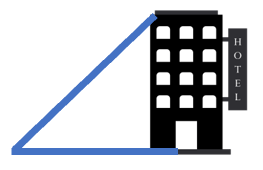# Math Worksheets Land

Math Worksheets For All Ages

# Math Worksheets Land

Math Worksheets For All Ages

Home > Math Topics > Trigonometry >

# Pythagorean Identities Worksheets

What Are Pythagorean Identities? Pythagorean identities are the true representation of Pythagoras theorem as functions. So, these identities can also help us in deciding fundamentally deciding the relationship between sine, cos and tan trigonometric functions. You can determine from that point the functions of other identities in our lives and how they can make things easy and simple too. Pythagorean identities are trigonometric identities, which are extensions of the Pythagorean theorem. The fundamental identities state that for every angle: sin2 Θ + cos2 Θ = 1 Pythagorean identities are extremely useful for simplifying trigonometric expressions, particularly in writing expressions as functions of either sine or cos in the statements of double angle formulas. There are there Pythagorean identities that you need to recall immediately. The first one is already mentioned above. The rest of the two are: 1 + cot2 Θ = csc2 Θ and 1 + tan2 Θ = sec2 Θ. Students can use these worksheets and lessons to learn techniques that help them determine missing value in trig. functions.

### Aligned Standard: HSF-TF.C.8

• Answer Keys - These are for all the unlocked materials above.
• Homework Worksheet - Write each expression in terms of a single trigonometric identity or a constant.
• Practice Worksheet - You will work through and simplify all of these as you go through this.

### How Pythagorean Identities Apply to the Real WorldA mathematical identity is an equation that holds true for all potential values. Pythagorean Identities are simply a series of statements that are true about trigonometric functions. They are all based on Pythagorean theorem, so hence the name. Their real function is to help us simplify trigonometric expressions. Most of these expressions we are putting together to find the length of the side or an angle measurement within a right triangle.

In the real world we use these identifies all of the time to help use better understand measures that matter to our daily routine. Say for instance that you needed to know how much scaffolding you needed to wash the windows of this hotel. You can calculate how tall it is by simply measuring your distance for the building and the angle at which you vision reaches the roof. That definitely could come in handy. This is one basic example, but it has endless applications in all forms of engineering and just about any form of construction whether it be for building structure or just simple geometric products.

Unlock all the answers, worksheets, homework, tests and more!
Save Tons of Time! Make My Life Easier Now

## Thanks and Don't Forget To Tell Your Friends!

I would appreciate everyone letting me know if you find any errors. I'm getting a little older these days and my eyes are going. Please contact me, to let me know. I'll fix it ASAP.